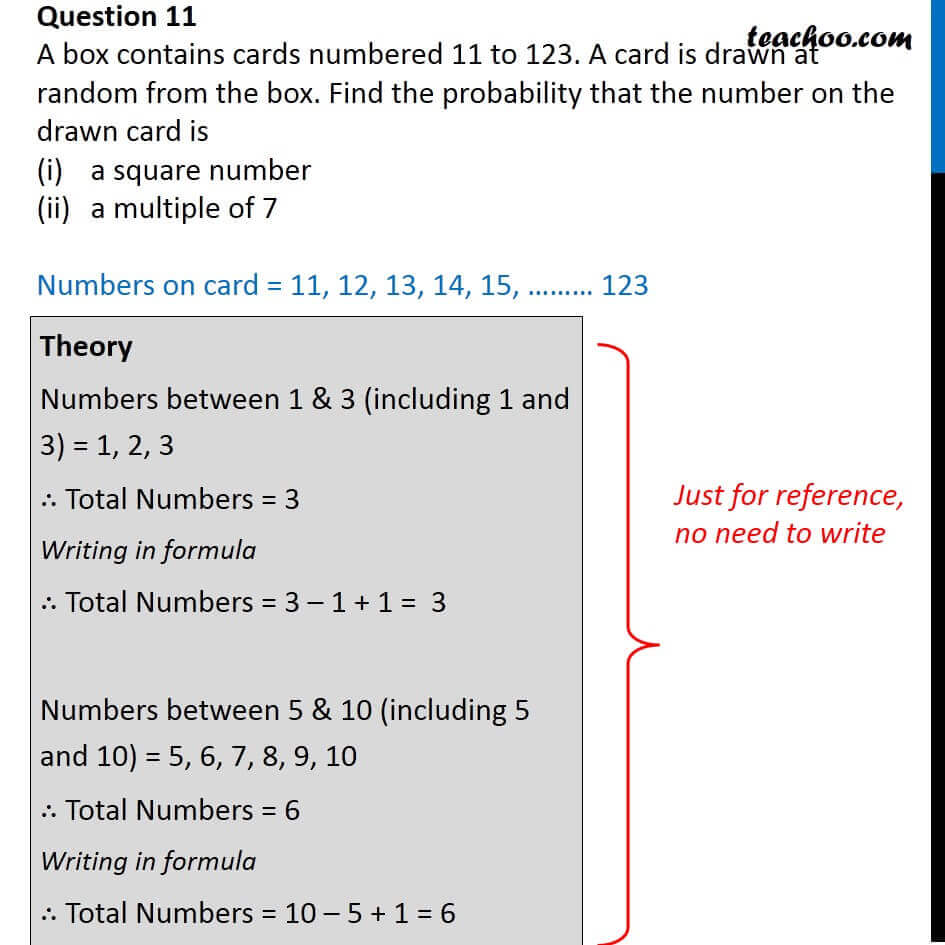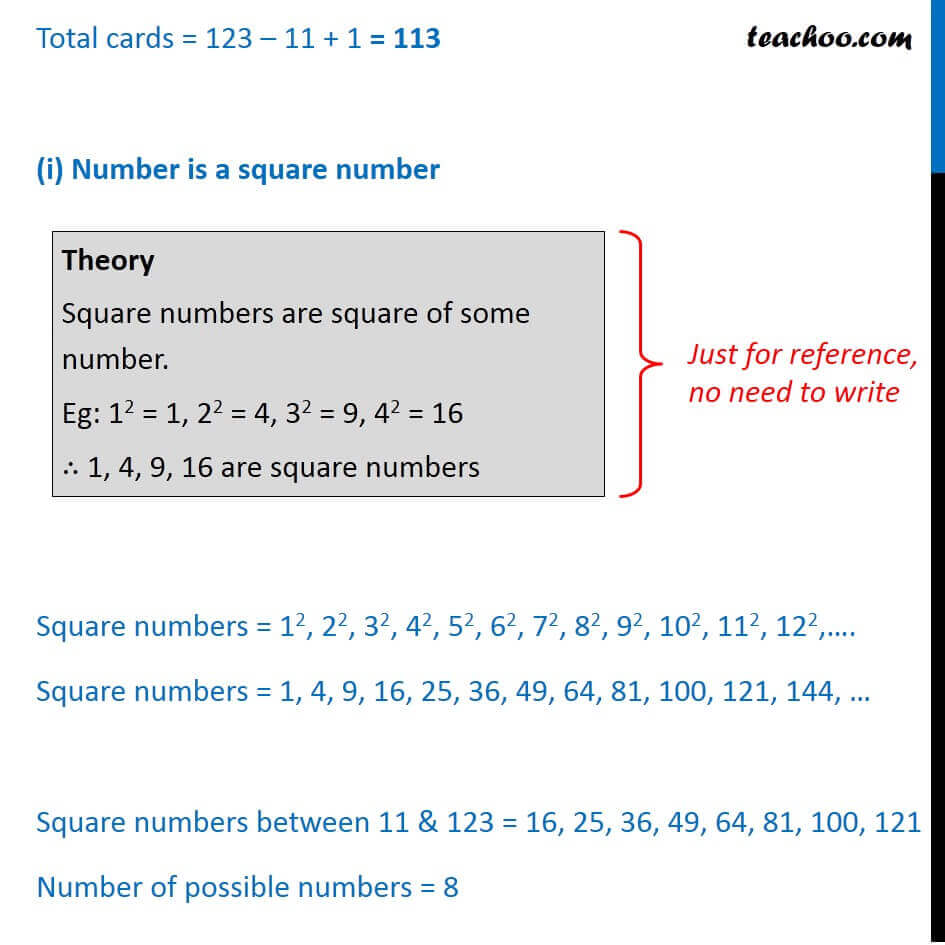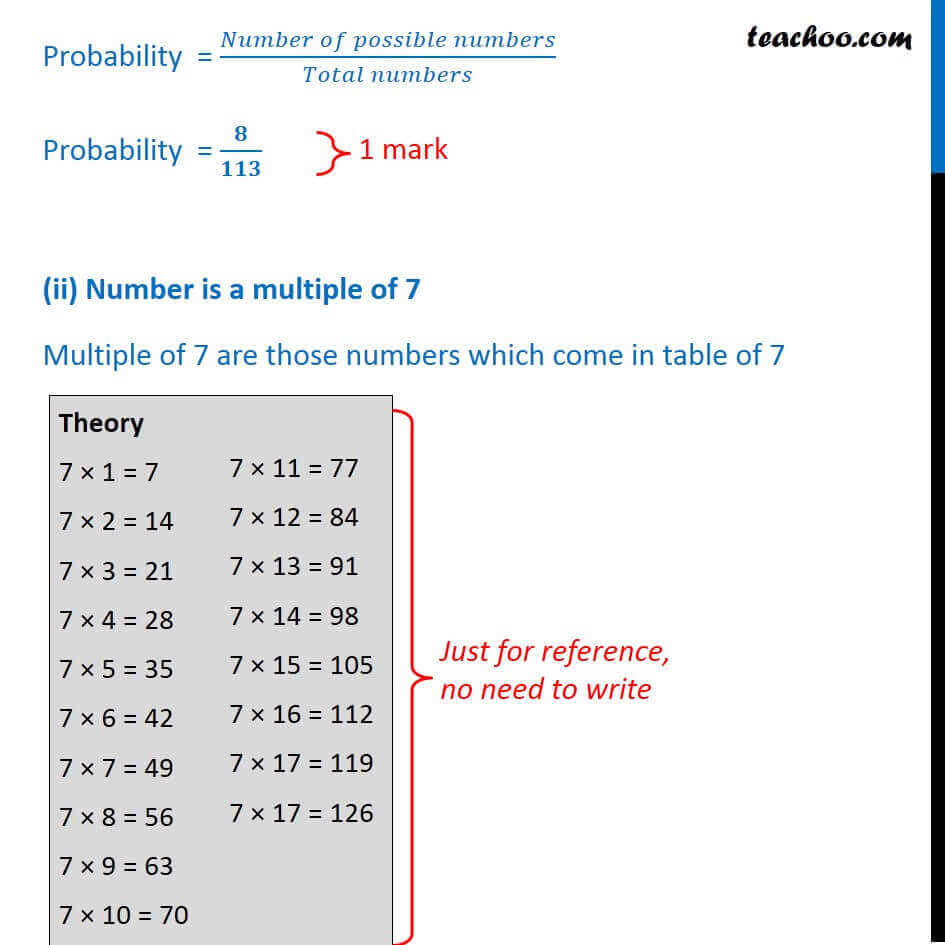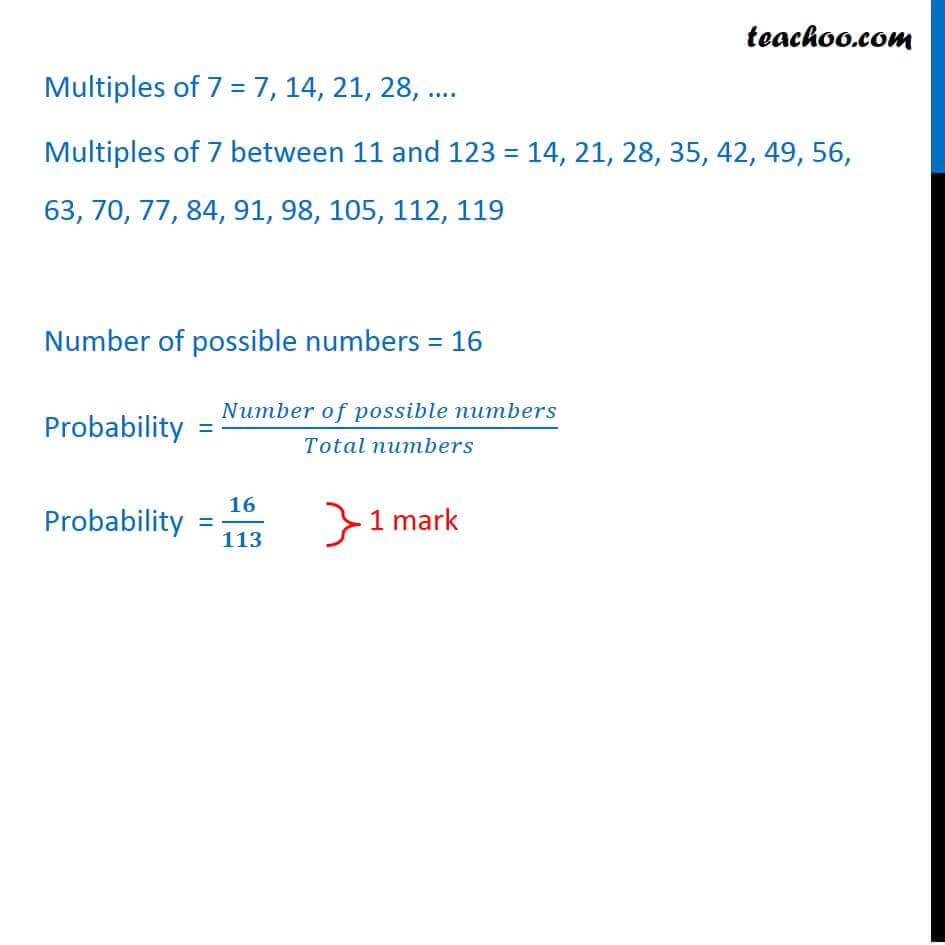CBSE Class 10 Sample Paper for 2018 Boards

Class 10
Solutions of Sample Papers for Class 10 Boards

### A box contains cards numbered 11 to 123. A card is drawn at random from the box. Find the probability that the number on the drawn card is

1. a square number
2. a multiple of 7

This is a question of CBSE Sample Paper - Class 10 - 2017/18.Learn in your speed, with individual attention - Teachoo Maths 1-on-1 Class

### Transcript

Question 11 A box contains cards numbered 11 to 123. A card is drawn at random from the box. Find the probability that the number on the drawn card is a square number a multiple of 7 Numbers on card = 11, 12, 13, 14, 15, ……… 123 Theory Numbers between 1 & 3 (including 1 and 3) = 1, 2, 3 ∴ Total Numbers = 3 Writing in formula ∴ Total Numbers = 3 – 1 + 1 = 3 Numbers between 5 & 10 (including 5 and 10) = 5, 6, 7, 8, 9, 10 ∴ Total Numbers = 6 Writing in formula ∴ Total Numbers = 10 – 5 + 1 = 6 Total cards = 123 – 11 + 1 = 113 (i) Number is a square number Square numbers = 12, 22, 32, 42, 52, 62, 72, 82, 92, 102, 112, 122,…. Square numbers = 1, 4, 9, 16, 25, 36, 49, 64, 81, 100, 121, 144, … Square numbers between 11 & 123 = 16, 25, 36, 49, 64, 81, 100, 121 Number of possible numbers = 8 Theory Square numbers are square of some number. Eg: 12 = 1, 22 = 4, 32 = 9, 42 = 16 ∴ 1, 4, 9, 16 are square numbers Probability = (𝑁𝑢𝑚𝑏𝑒𝑟 𝑜𝑓 𝑝𝑜𝑠𝑠𝑖𝑏𝑙𝑒 𝑛𝑢𝑚𝑏𝑒𝑟𝑠)/(𝑇𝑜𝑡𝑎𝑙 𝑛𝑢𝑚𝑏𝑒𝑟𝑠) Probability = 𝟖/𝟏𝟏𝟑 (ii) Number is a multiple of 7 Multiple of 7 are those numbers which come in table of 7 Theory 7 × 1 = 7 7 × 2 = 14 7 × 3 = 21 7 × 4 = 28 7 × 5 = 35 7 × 6 = 42 7 × 7 = 49 7 × 8 = 56 7 × 9 = 63 7 × 10 = 70 7 × 11 = 77 7 × 12 = 84 7 × 13 = 91 7 × 14 = 98 7 × 15 = 105 7 × 16 = 112 7 × 17 = 119 7 × 17 = 126